Math Puzzle
Logic Puzzle
Trigonometry
Fun Facts
100

A blueberry cheesecake meant for dessert was already half-eaten in the afternoon. Below are the children's replies when their mom checked with them

Robert: Sarah ate the cake

Sarah: Robert lied

Tony: Come on! I didn't even open the fridge.

Only one of them told the truth. Who ate the cake?

Tony

(Sarah is telling the truth and Tony did open the fridge)

100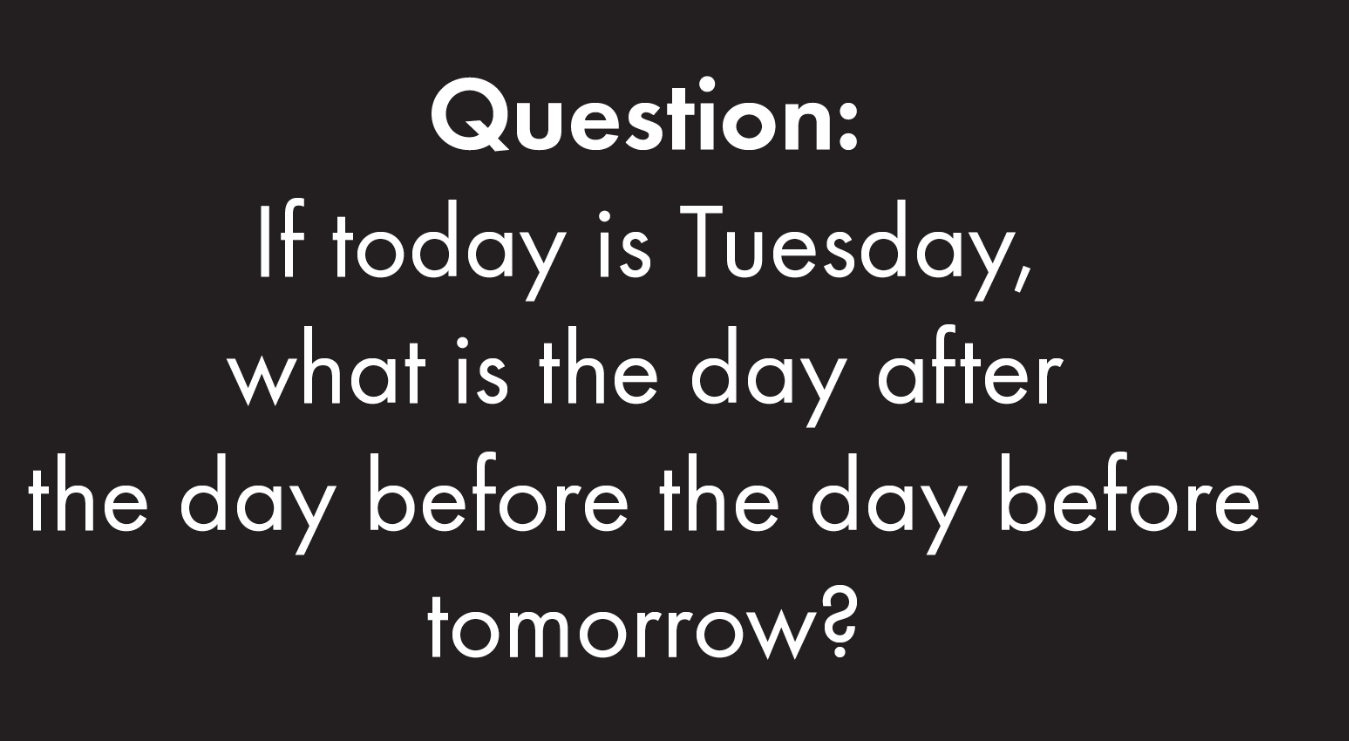Tuesday

100

In the triangle ABC, AB = BC = 29, and AC = 42. What is the area of the triangle ABC?

420

Height is

sqrt(29^2-21^2)

100

What is the current world population?

200

In a room with 8 people, everyone shakes hands with everybody else exactly once. How many handshakes are there?

(8*7)/2=28

200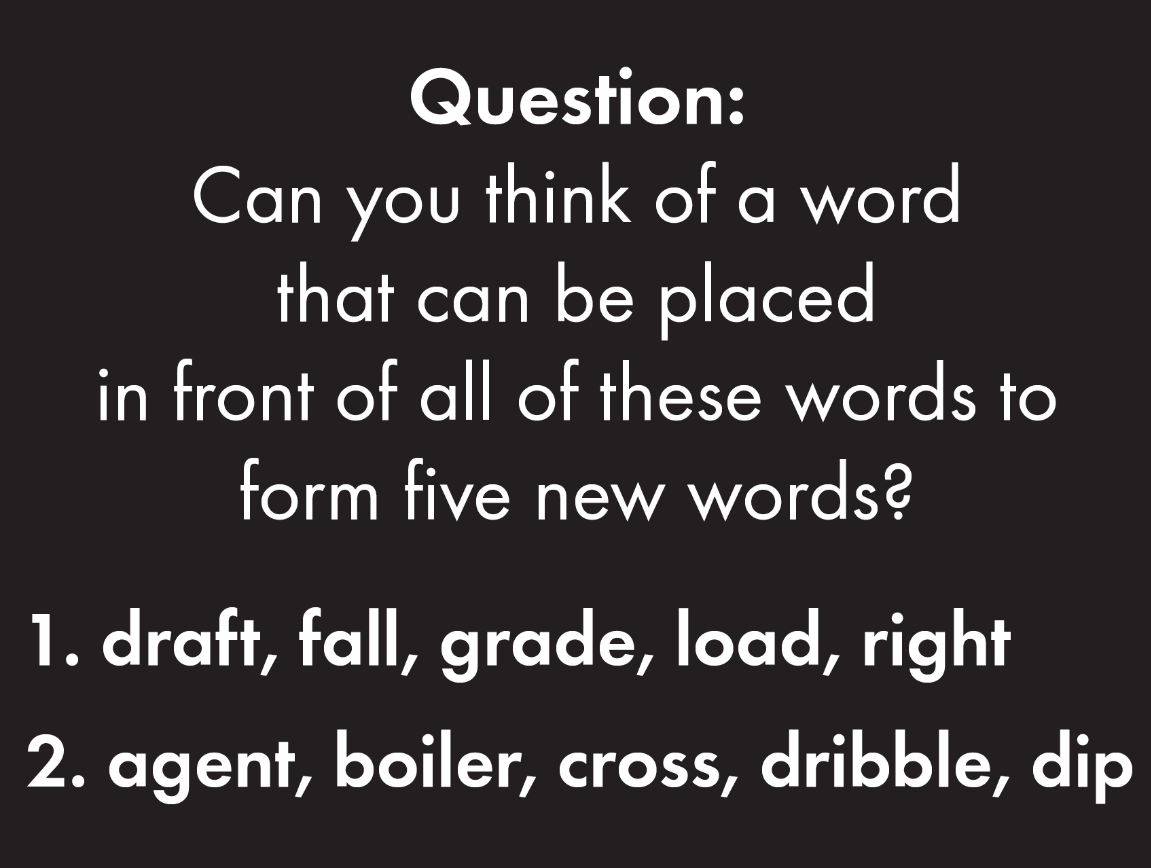1) Down

2) Double

200

If all 4 circles are equal and the radius is 6 inches, what is the area of the shaded region? Leave your answer in terms of pi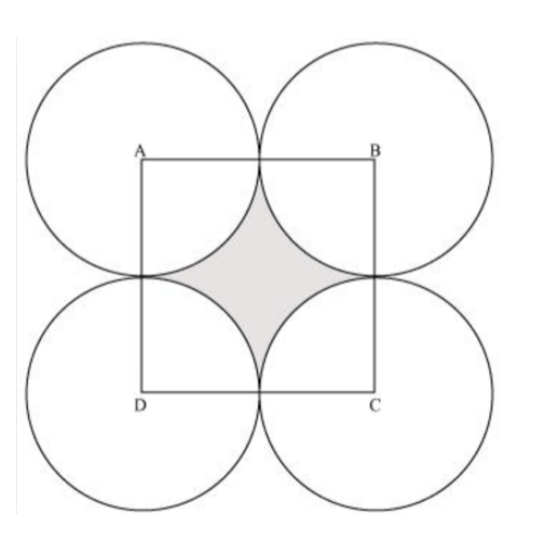144 - 36 pi

200
How many Earths can fit inside the sun?

(FYI, the diameter of Earth is about 8,000 miles)

1.3 million

300

I phoned my mom to help me answer this, the final question on a quiz show: How many integers equal their own squares? Mon said _?__. She was right!

Two

(Zero and One)

300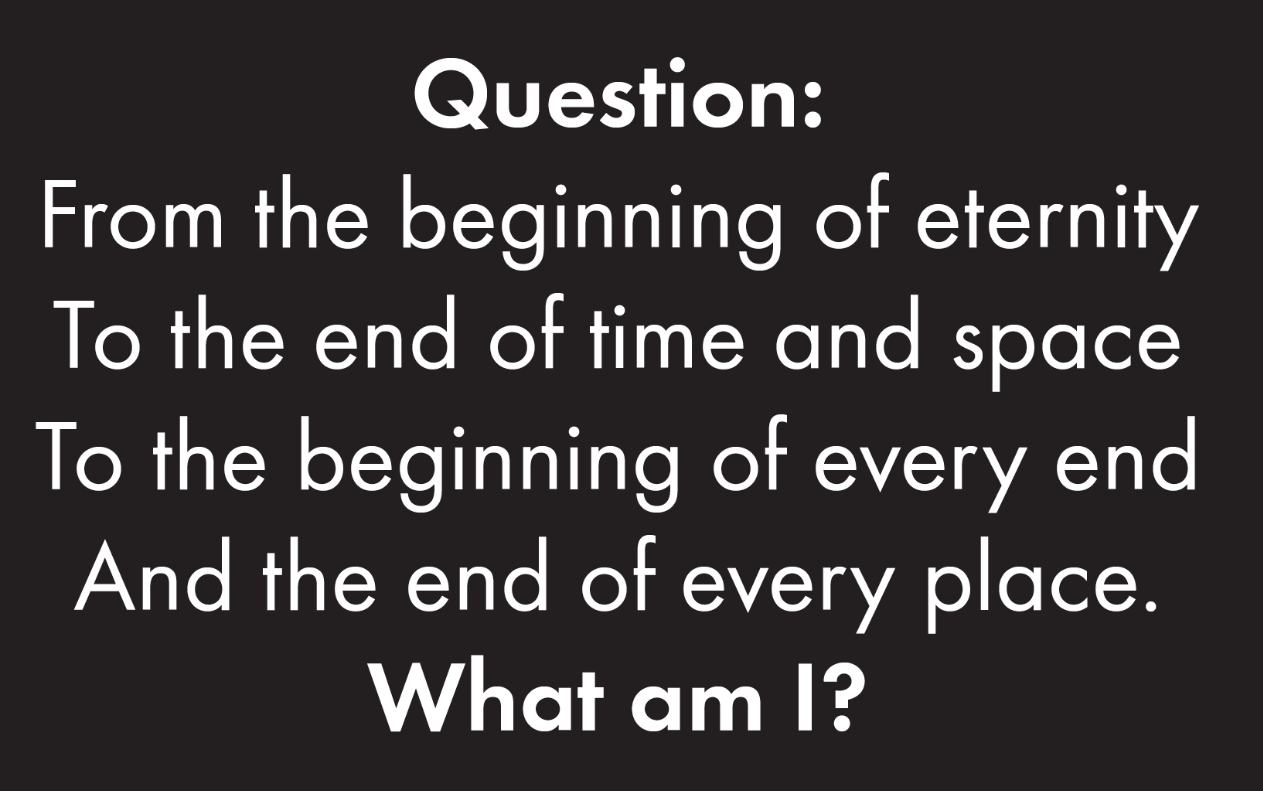The Letter E

300

In the given regular hexagon, ABJI and FEHG are squares with areas of 18 and 32 respectively. JBK is equilateral and FE = BC.

What is the area of KBC?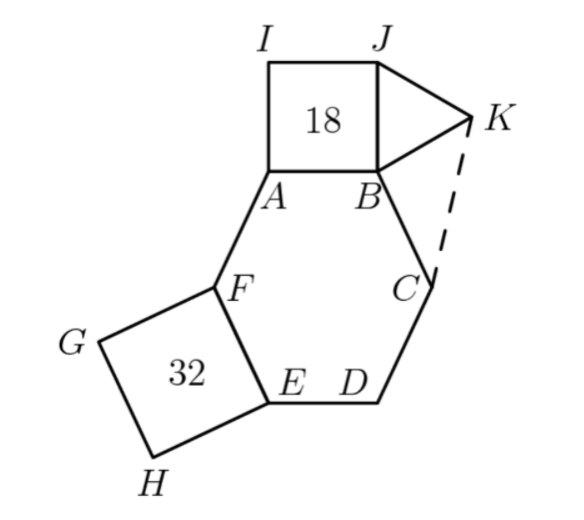12

300

What is the name of the division sign?

Obelus

400

What is the ten' digit of the product

1 * 2 * 3 * ... * 98 * 99?

0

400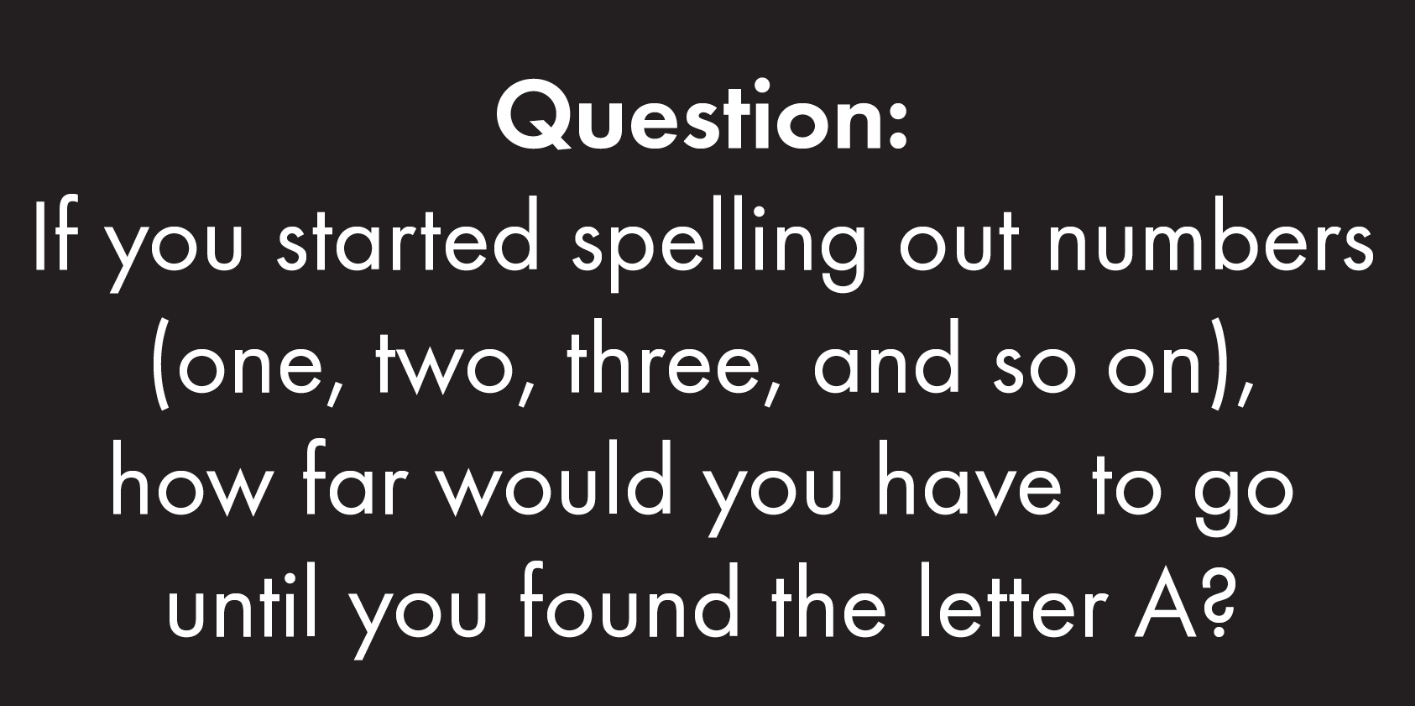One Thousand

400

A large equilateral triangle is formed from 64 small equilateral triangles, each having a side of 3. Find the perimeter of the large equilateral triangle.

one side is 24, so the perimeter is 72400

How many people do we need to have in a room, so that there's a 50% chance that two people have the same birthday?

23

500

A 600-pound pumpkin was entered in a contest. When it arrived, it was 99% water. The pumpkin sat for days in the hot sun, lost some weight (water only) and is now 98.5% water. How much does it weigh?

400 pounds

500e, n, t

(eight, nine, ten)

500

A semicircle is inscribed in an isosceles triangle with base 16 and height 15 so that the diameter of the semicircle is contained in the base of the triangle as shown. What is the radius of the semicircle?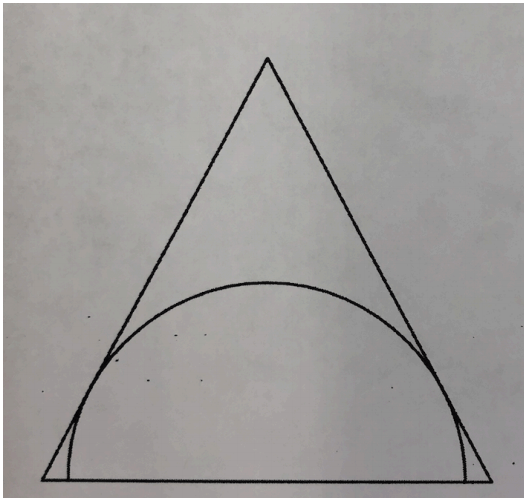r * 17 = 15 * 8

r=120/7

500

What is the world record for pi memorization?

Sharman, Suresh Kuma from India memorized 70,030 digits. It took him 17 hours 14 min.

Click to zoom